Category: Reverse Engineering Points: 100 Solves: Description:

ZorroPub

zorro_bin

First off, lets see what type of file this is:

`\$ file zorro_binzorro_bin: ELF 64-bit LSB executable, x86-64, version 1 (SYSV), dynamically linked (uses shared libs), for GNU/Linux 2.6.24, BuildID[sha1]=5bd9436f341615c804471bb5aec37e426508a7af, stripped`

Standard issue x64 Linux binary. Next, lets try running it:

`\$ ./zorro_bin Welcome to Pub Zorro!!Straight to the point. How many drinks you want?1  OK. I need details of all the drinks. Give me 1 drink ids:12345Looks like its a dangerous combination of drinks right there.Get Out, you will get yourself killed`

So it takes two inputs from the command line. The first appears to indicate the number of inputs you will be putting in the second. Running strings doesn't reveal too much, but it does show what our winning text is.

`You choose right mix and here is your reward: The flag is nullcon{%s}`

I did try running ltrace on it, but really nothing special came out of that. Next, let's open it up in IDA. The following are the major points of it's control flow.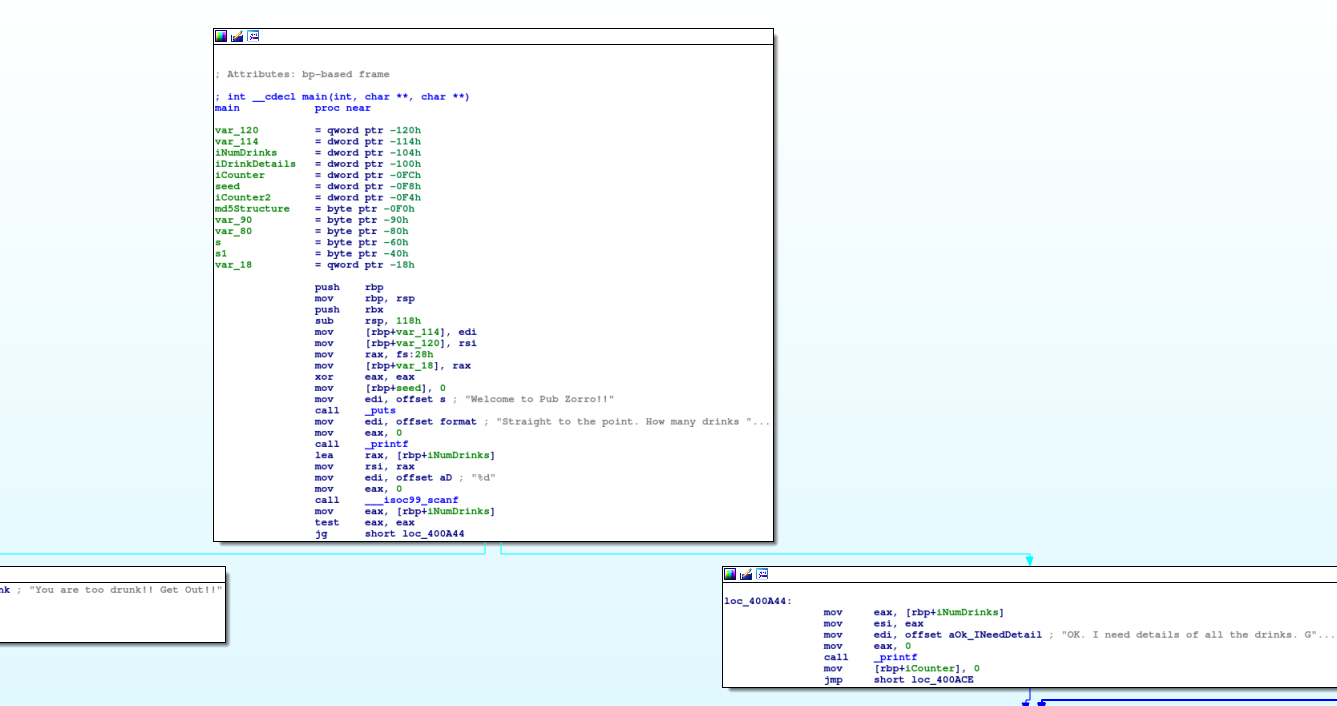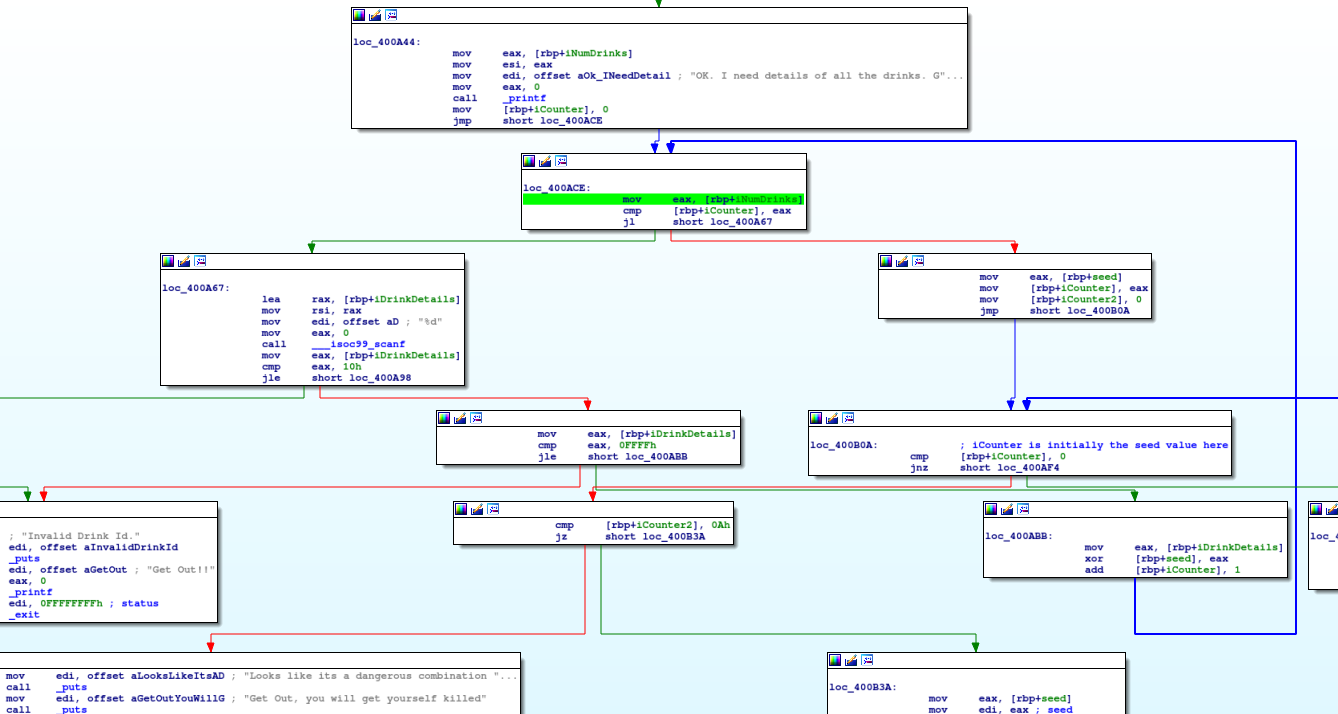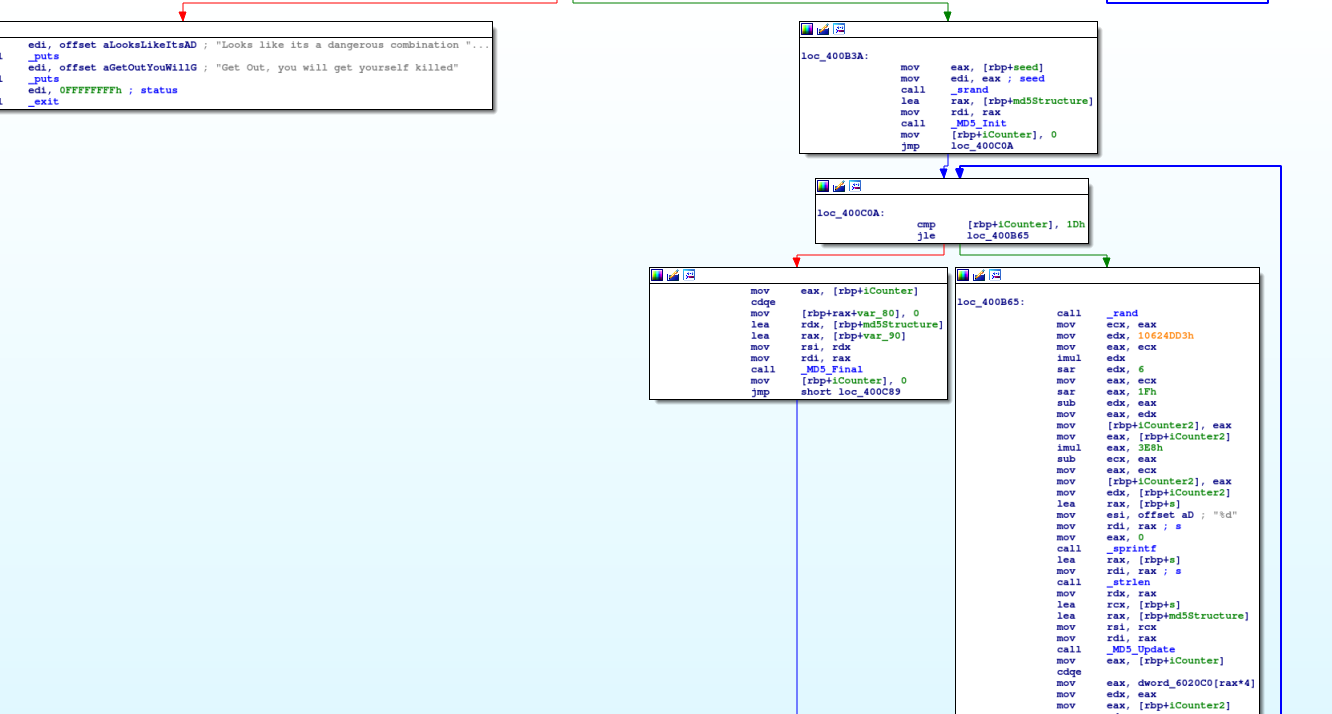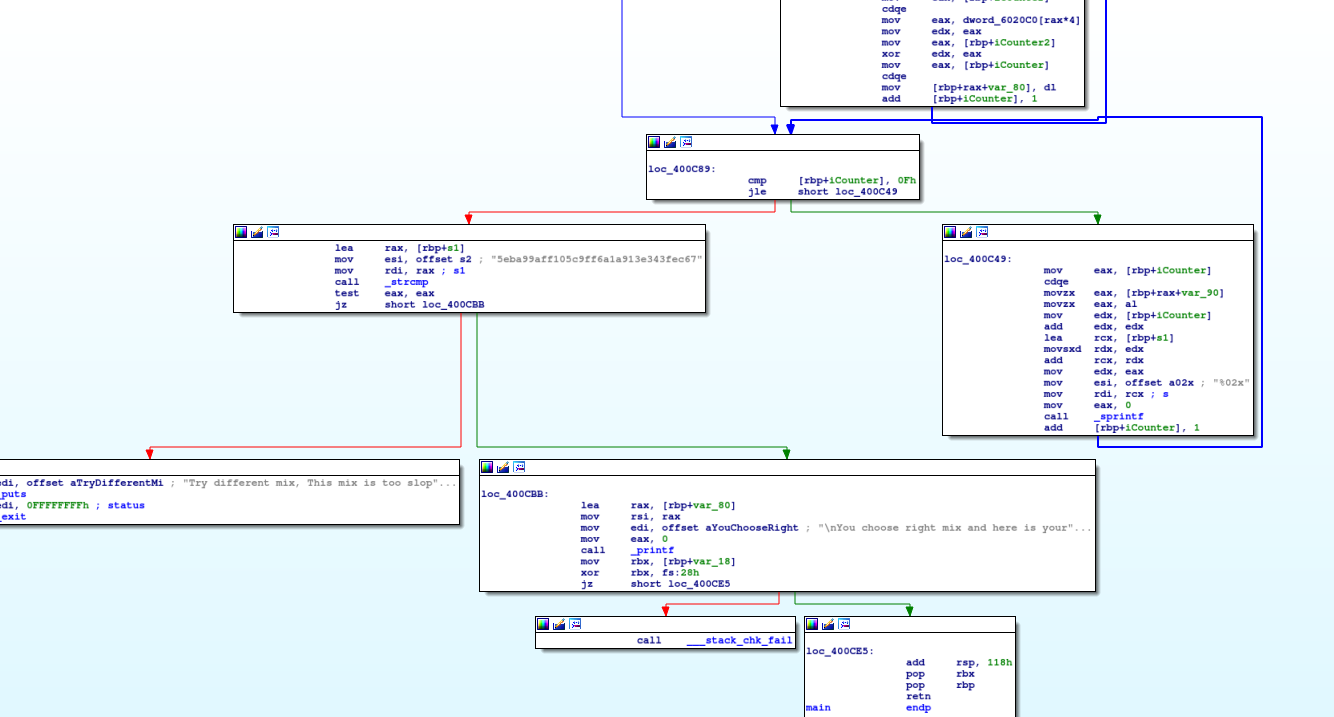In situations like this, it is often helpful to translate the assembly into a language that you can more easily understand. In my case, I went ahead an translated this into Python (partially). In the first part, we can see that this program basically puts some text to the screen then asks for a %d (signed integer) input. This can be done as follows:

`#!/usr/bin/python3print("Welcome to Pub Zorro!")numDrinks = int(input("Straight to the point. How many drinks you want? "),10)`

Next, we see the following checks:

`call    ___isoc99_scanfmov     eax, [rbp+iNumDrinks]test    eax, eaxjg      short loc_400A44`

This is checking that the number of drinks you want is more than 0. So, in python:

`if numDrinks <= 0:    print("You are too drunk!! Get Out!!")    exit(1)`

Let's take a look at the next section: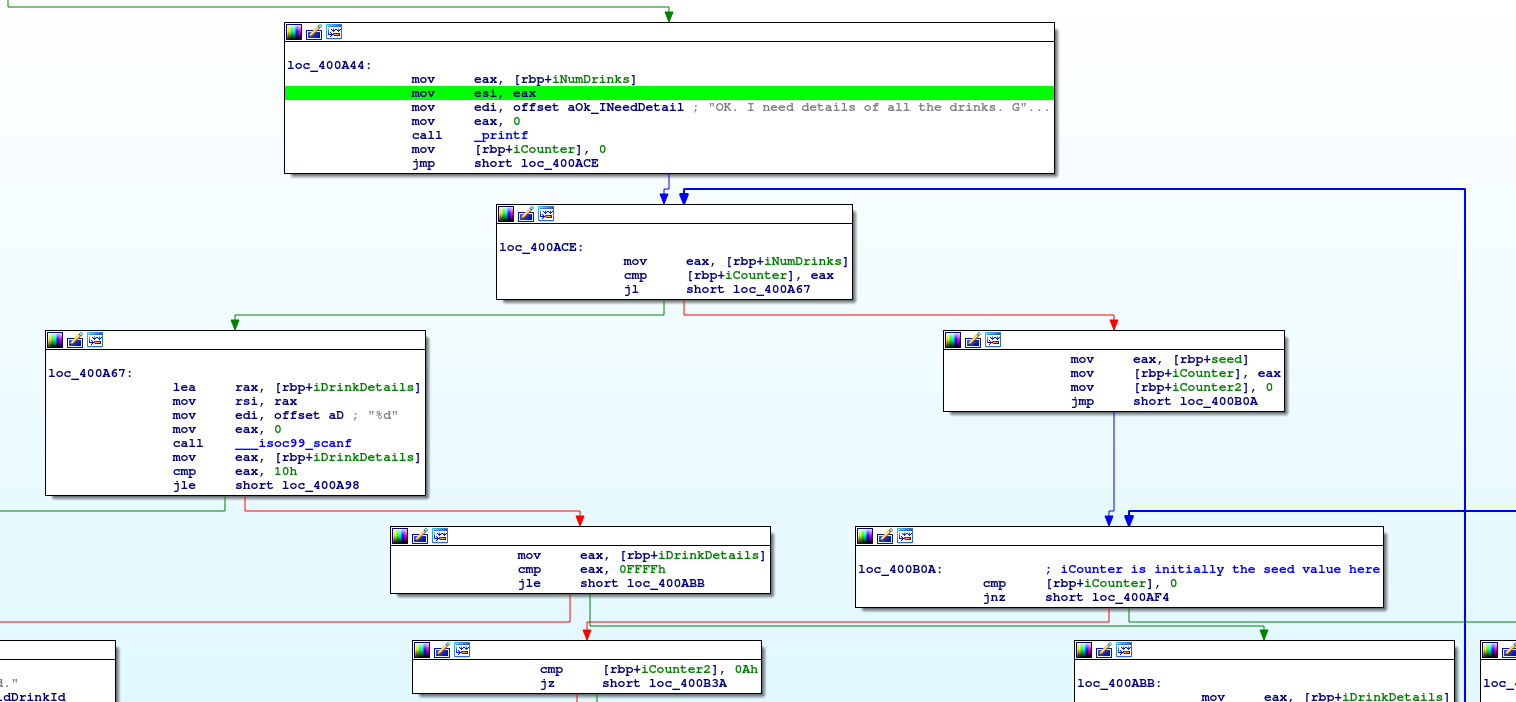loc_400A44 is just printing out our next prompt and setting a counter to 0.

loc_400ACE is comparing the number of drinks we said we would have, with the counter we just set to 0. So this means we're going to be looping one by one through each drink.

loc_400A67 is called for each drink. It calls scanf to get the integer input for the drink. It then compares it with "10h" which is 16 and errors out if the integer is less than or equal to it. It the performs the follow on check if the input is less than or equal to "ffffh" which is 65535. So this means that our "drink details" is an integer between 17 and 65535 inclusive.

Let's now take a look at the right side of that loop: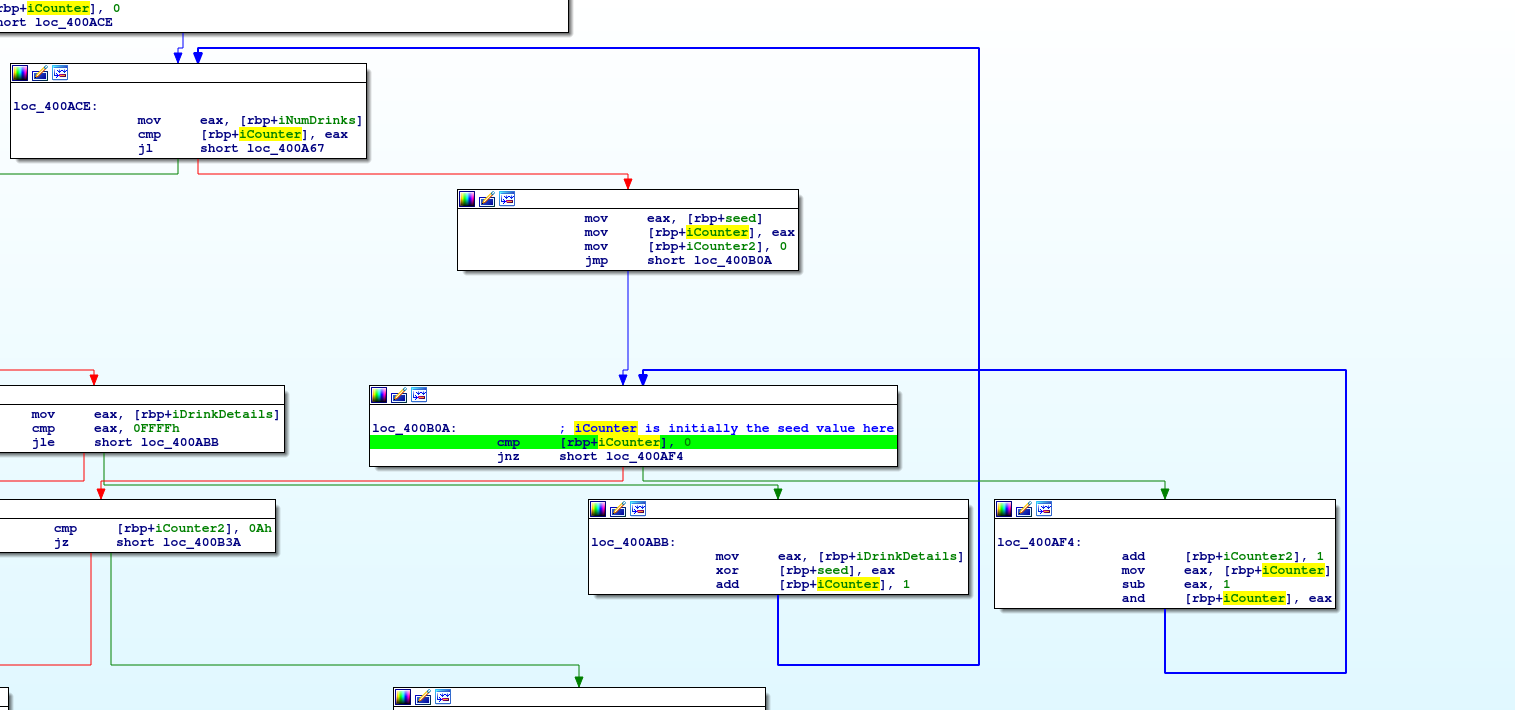Once we have validated the drink input, execution goes to loc_400ABB. Here is where we actually do something with it. We xor this new value with the seed (initially zero). Put all together, we get the following:

`seed = 0for drinkID in input("OK. I need details of all the drinks. Give me %d drink ids: ").split(" "):    drinkID = int(drinkID,10)    if drinkID <= 16 or drinkID > 65535:        print("Invalid Drink Id.")        print("Get Out!!")        exit(1)    # Update the seed    seed ^= drinkID`

So we can see that we are just combining all the drink inputs together. Next, we do:

`mov     eax, [rbp+seed]mov     [rbp+iCounter], eaxmov     [rbp+iCounter2], 0jmp     short loc_400B0A`

This is equivalent to:

`iCounter = seediCounter2 = 0`

We find ourself in a loop between loc_400B0A and loc_400AF4. The instructions are:

```cmp     [rbp+iCounter], 0jnz     short loc_400AF4
add     [rbp+iCounter2], 1mov     eax, [rbp+iCounter]sub     eax, 1and     [rbp+iCounter], eax```

We're comparing our counter to zero, remembering that we just previously set it to our calculated seed value. If it's not zero, then we increment our loop counter (counter2) then and the value with itself minus 1. In this way, we are looping until the first time that our and value hits zero.

`while iCounter != 0:    iCounter2 += 1    iCounter &= iCounter - 1`

At this point, our iCounter is equal to zero, and our iCounter2 is the number of and operations it took to get there.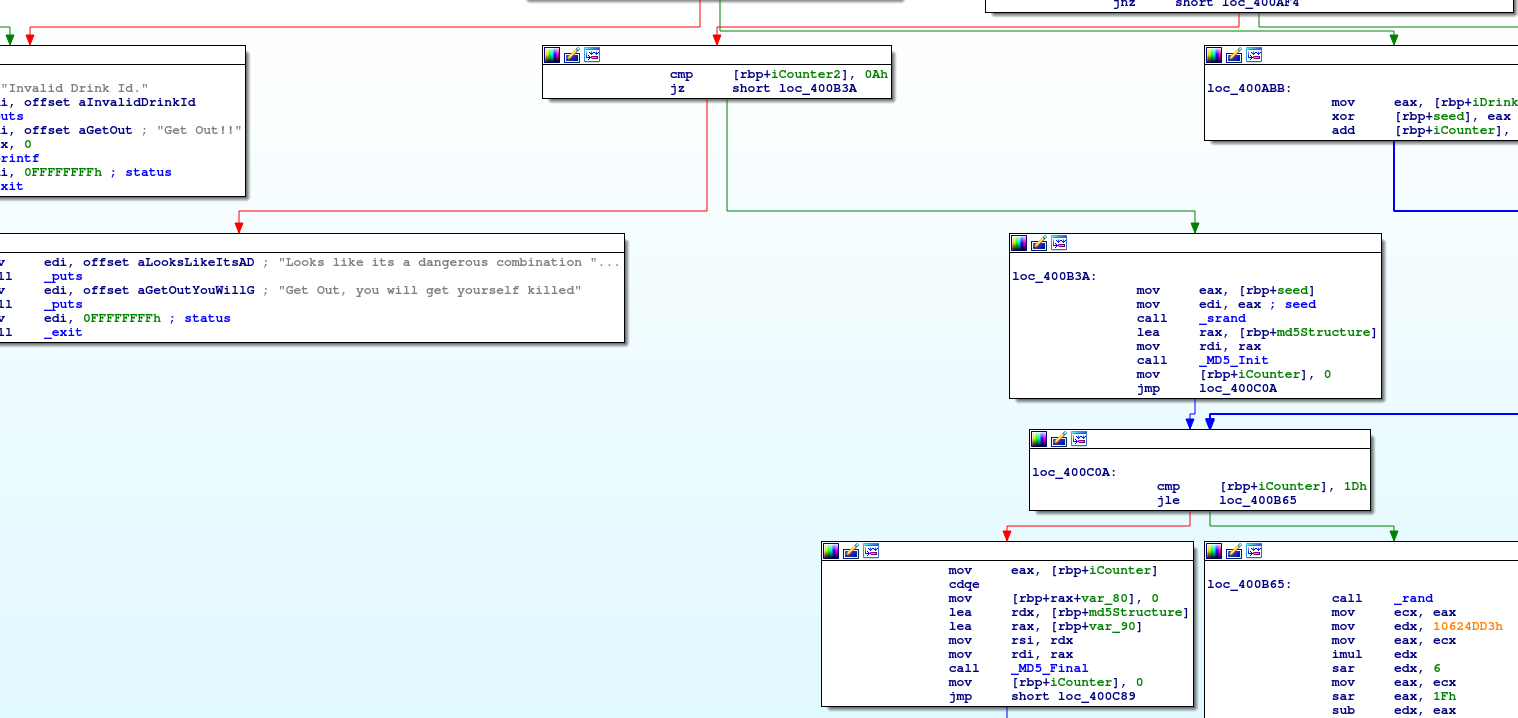We can see right in the upper middle there is one final check before we get to the next section. It's comparing the counter with 10. This means that the values we put in must be able to get through the and operations in exactly 10 loops. After this, we go on to some more complicated interactions including hashes and stuff. Here's what we've got so far:

`#!/usr/bin/python3print("Welcome to Pub Zorro!")numDrinks = int(input("Straight to the point. How many drinks you want? "),10)if numDrinks <= 0:    print("You are too drunk!! Get Out!!")    exit(1)seed = 0for drinkID in input("OK. I need details of all the drinks. Give me %d drink ids: ").split(" "):    drinkID = int(drinkID,10)    if drinkID <= 16 or drinkID > 65535:        print("Invalid Drink Id.")        print("Get Out!!")        exit(1)    # Update the seed    seed ^= drinkIDiCounter = seediCounter2 = 0while iCounter != 0:    iCounter2 += 1    iCounter &= iCounter - 1if iCounter2 != 10:    print("Looks like its a dangerous combination of drinks right there.")    print("Get Out, you will get yourself killed")    print("debug: counter is {0}".format(iCounter2))    exit(1)`

At this point, we can scrutinize the control flow more easily. Because xor is an operation that just maps onto itself, and because there's no other use of the input values, we can safely assume that we really only need one drink of the correct value. More than one drink would simply be creating the same value.  Also, notice that bounds of this value are between 17 and 65535. The implications here is that our entire search space is roughly 65500 values. This is TINY. We can effectively brute force the correct answer by just trying all of the values. To do so, I used a bash one-liner:

`for i in \$(seq 1 65535); do echo -e "1\n\$i" | ./zorro_bin | grep -i nullcon ; done`

This says loop through 1-65535 (yeah, you could set it to 7), echo the input as command-line input to the zorro_bin application, then find the winner by looking for the unique string "nullcon". Doing so nets us the key.

`flag: nullcon{nu11c0n_s4yz_x0r1n6_1s_4m4z1ng}`